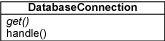PHP设计模式 之 单元素模式

# PHP设计模式 之 单元素模式

``````<?php
require_once("DB.php");

class DatabaseConnection
{
public static function get()
{
static \$db = null;
if (\$db == null)
\$db = new DatabaseConnection();
return \$db;
}

private \$_handle = null;

private function __construct()
{
\$this->_handle = &DB::Connect(\$dsn, array());
}

public function handle()
{
return \$this->_handle;
}
}

print("Handle = " . DatabaseConnection::get()->handle() . "\n");
print("Handle = " . DatabaseConnection::get()->handle() . "\n");````````````% php singleton.php
Handle = Object id #3
Handle = Object id #3
%``````